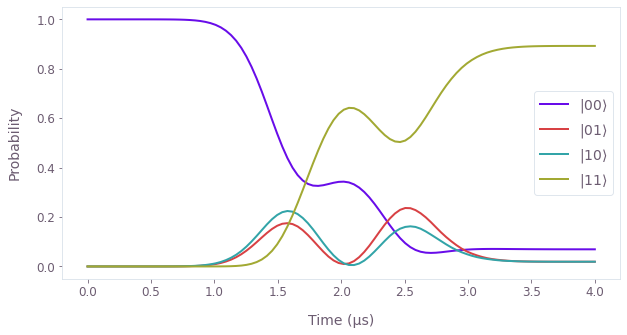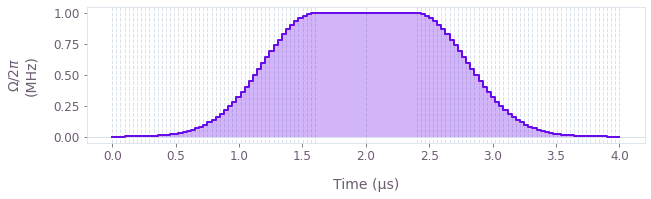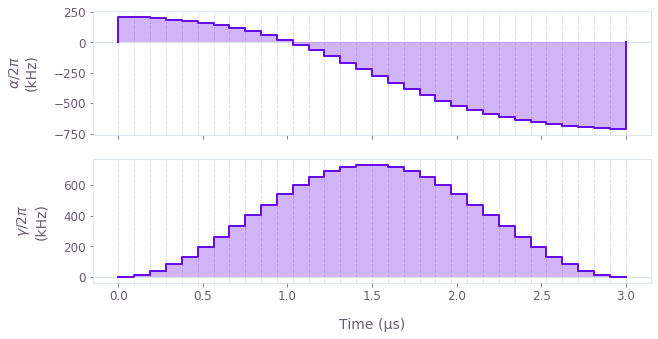# How to create analytical pulses for simulation and optimization

Use predefined pulses from the Boulder Opal toolkit

The Boulder Opal toolkit contains analytical pulses in the form of piecewise-constant functions (Pwcs), which can be used for simulation and optimization. The full list of Pwc analytical pulses in the toolkit is available in the reference documentation.

The Boulder Opal Toolkits are currently in beta phase of development. Breaking changes may be introduced.

All pulses require a duration to be passed, with the pulses defined from time zero to time duration. Most of the pulses also require segment_count to be defined, which determines the number of segments in the Pwc. Many of the parameters of the pulses can be passed as tensors and thus can be optimized to minimize a given cost function.

In this notebook we show how to create pulses from the toolkit for use in simulation and optimization. You can also define your own analytical Hamiltonians from the ground up, see this user guide for more information.

## Summary workflow

### 1. Create a node defining the pulse

Once a graph has been set up for the system, create a Pwc analytic pulse using one of the pulses from the toolkit. If one is running an optimization problem, define the optimizable pulse parameters using graph.optimization_variable, before defining the pulse. Then add the pulse (multiplied by the appropriate operator) to the Hamiltonian.

### 2. Execute the graph for the simulation/optimization

With the graph object created, a simulation can be run using the qctrl.functions.calculate_graph function. The outputs and the graph must be provided. The function returns the results of the simulation.

Alternatively, an optimization can be run using the qctrl.functions.calculate_optimization function or the qctrl.functions.calculate_stochastic_optimization function. The cost, the outputs, and the graph must be provided. The function returns the results of the optimization.

## Worked example: Simulation with analytical pulses

In this example, we will simulate two interacting qubits (labeled 0 and 1), with one of the qubits (qubit 0) driven by an external pulse.

The Hamiltonian for the system is \begin{equation} H(t) = \frac{1}{2} \left(\Omega(t) \sigma^{-}_{0} + \Omega(t)^* \sigma^{+}_{0} \right) + \sum_{i=x,y,z} \delta_i \sigma^{i}_{0} \sigma^{i}_{1} \,, \end{equation} where $\Omega(t)$ is the external pulse and $\delta_i$ is the qubit coupling.

The pulse used for this example is the Gaussian pulse, graph.pulses.gaussian_pulse_pwc, defined as \begin{equation}\Omega(t) = \begin{cases} A \exp \left(- \frac{(t-t_1)^2}{2\sigma^2} \right) &\mathrm{if} \quad t < t_1=t_0- t_\mathrm{flat}/2 \\ A &\mathrm{if} \quad t_0-t_\mathrm{flat}/2 \le t < t_0+t_\mathrm{flat}/2 \\ A \exp \left(- \frac{(t-t_2)^2}{2\sigma^2} \right) &\mathrm{if} \quad t > t_2=t_0+t_\mathrm{flat}/2 \end{cases} \, , \end{equation} where $A$ is the amplitude, $\sigma$ is the width of the Gaussian, $t_0$ is the center of the pulse, and $t_\mathrm{flat}$ is the flat duration.

import matplotlib.pyplot as plt
import numpy as np
from qctrl import Qctrl
from qctrlvisualizer import get_qctrl_style, plot_controls, plot_populations

# Starting a session with the API.
qctrl = Qctrl()

plt.style.use(get_qctrl_style())

# Create the data flow graph describing the system.
graph = qctrl.create_graph()

# Define the duration of the pulse.
duration = 4e-6  # s

# Define the parameters of the pulse.
pulse = graph.pulses.gaussian_pulse_pwc(
duration=duration,
segment_count=100,
amplitude=2 * np.pi * 1.0e6,  # rad/s
width=duration * 0.1,  # s
flat_duration=duration * 0.2,  # s
name="$\Omega$",
)

# Add the pulse terms to the Hamiltonian.
hamiltonian = graph.hermitian_part(
pulse * graph.pauli_kronecker_product([("M", 0)], subsystem_count=2)
)

# Add the qubit-qubit coupling terms to the Hamiltonian.
couplings = {"X": 8.0e6, "Y": 8.0e6, "Z": 9.0e6}  # rad/s

for i, coupling in couplings.items():
hamiltonian += coupling * graph.pauli_kronecker_product(
[(i, 0), (i, 1)], subsystem_count=2
)

sample_times = np.linspace(0.0, duration, 100)

# Compute the unitary using time_evolution_operators_pwc.
unitaries = graph.time_evolution_operators_pwc(
hamiltonian=hamiltonian, sample_times=sample_times
)

# Define the initial state.
initial_state = graph.fock_state(4, 0)[:, None]

# Evolve the initial state with the unitary to get the final state.
evolved_states = unitaries @ initial_state
evolved_states.name = "states"

# Run simulation.
result = qctrl.functions.calculate_graph(
graph=graph, output_node_names=["$\Omega$", "states"]
)

Your task calculate_graph (action_id="1152888") has completed.

# Plot the evolution of the populations of the qubits using plot_populations.
states = result.output.pop("states")
qubit_populations = np.abs(states["value"].squeeze()) ** 2

plot_populations(
plt.figure(),
sample_times,
{rf"$|{k:02b}\rangle$": qubit_populations[:, k] for k in range(4)},
)# View the generated Gaussian pulse using the plot_controls function.
plot_controls(plt.figure(), result.output)## Worked example: Optimization with analytical pulses

In this example, we will implement an X gate in a noisy single-qubit system, by optimizing the parameters of two pulses.

The Hamiltonian for the system is \begin{equation} H(t) = \alpha(t) \sigma_{z} + \frac{1}{2}\left(\gamma(t)\sigma_{-} + \gamma^*(t)\sigma_{+}\right) + \delta \sigma_{z} + \zeta(t) \sigma_{z} \,, \end{equation} where $\alpha(t)$ and $\gamma(t)$ are pulses with optimizable parameters, $\delta$ is the qubit detuning, and $\zeta(t)$ is a dephasing noise process.

The $\alpha(t)$ pulse acting on $\sigma_{z}$ is a hyperbolic tangent pulse, graph.pulses.tanh_ramp_pwc, defined by \begin{equation} \alpha(t) = \frac{a_+ + a_-}{2} + \frac{a_+ - a_-}{2} \tanh\left( \frac{t - t_0}{t_\mathrm{ramp}} \right) \; , \end{equation} where $a_\pm$ are the asymptotic values of the pulse, $t_0$ is the center time, and $t_\mathrm{ramp}$ is the characteristic duration of the pulse.

The $\gamma(t)$ pulse acting on $\sigma_{-}$ is a cosine pulse, graph.pulses.cosine_pulse_pwc, defined by \begin{equation} \gamma(t) = \frac{A}{2} \left[1 + \cos \left(\frac{2\pi}{\tau} t- \pi \right)\right] = A \sin^2 \left(\frac{\pi}{\tau}t\right)\; , \end{equation} where $A$ is the amplitude and $\tau$ is the given duration of the pulse.

graph = qctrl.create_graph()

# Define the duration of the pulses.
duration = 3e-6  # s

# Define the number of segments for Pwc pulses.
segment_count = 32

# Define α tanh pulse acting on sigma_z.
alpha_start_value = graph.optimization_variable(
count=1, lower_bound=-3e7, upper_bound=3e7, name="alpha_start_value"
)
alpha_end_value = graph.optimization_variable(
count=1, lower_bound=-3e7, upper_bound=3e7, name="alpha_end_value"
)
alpha_ramp_duration = graph.optimization_variable(
count=1, lower_bound=0.1e-6, upper_bound=duration, name="alpha_ramp_duration"
)
alpha_center_time = graph.optimization_variable(
count=1, lower_bound=0.0, upper_bound=duration, name="alpha_center_time"
)
alpha_pulse = graph.pulses.tanh_ramp_pwc(
duration=duration,
segment_count=segment_count,
start_value=alpha_start_value,
end_value=alpha_end_value,
ramp_duration=alpha_ramp_duration,
center_time=alpha_center_time,
name=r"$\alpha$",
)

# Define γ cosine pulse acting on sigma_-.
gamma_amplitude = graph.optimization_variable(
count=1,
lower_bound=-2.0 * np.pi * 1e6,
upper_bound=2.0 * np.pi * 1e6,
name="gamma_amplitude",
)
gamma_pulse = graph.pulses.cosine_pulse_pwc(
duration=duration,
segment_count=segment_count,
amplitude=gamma_amplitude,
name=r"$\gamma$",
)

# Create pulse Hamiltonian.
hamiltonian = alpha_pulse * graph.pauli_matrix("Z")
hamiltonian += graph.hermitian_part(gamma_pulse * graph.pauli_matrix("M"))

delta = 2 * np.pi * 0.25e6  # rad/s
hamiltonian += delta * graph.pauli_matrix("Z")

# Define target gate.
target = graph.target(operator=graph.pauli_matrix("X"))

zeta = 2 * np.pi * 20e3  # rad/s
dephasing = zeta * graph.pauli_matrix("Z")

# Robust infidelity.
robust_infidelity = graph.infidelity_pwc(
hamiltonian=hamiltonian,
noise_operators=[dephasing],
target=target,
name="robust_infidelity",
)

# Compute the unitary using time_evolution_operators_pwc.
unitary = graph.time_evolution_operators_pwc(
hamiltonian=hamiltonian, sample_times=np.array([duration]), name="unitary"
)

result = qctrl.functions.calculate_optimization(
graph=graph,
cost_node_name="robust_infidelity",
output_node_names=[
"alpha_start_value",
"alpha_end_value",
"alpha_ramp_duration",
"alpha_center_time",
"gamma_amplitude",
"unitary",
r"$\alpha$",
r"$\gamma$",
],
)

print(f"Optimized cost: {result.cost:.3e}")

Your task calculate_optimization (action_id="1152889") has completed.
Optimized cost: 1.965e-12

# Check that the unitary operator associated with the evolution of the system Hamiltonian
# does indeed correspond to an X gate.
result.output.pop("unitary")["value"].round(5)

array([[[-0.-0.j, -0.-1.j],
[ 0.-1.j, -0.+0.j]]])

# Print out the converged pulse parameters.
print("Alpha Pulse")
for param in ["start_value", "end_value"]:

for param in ["ramp_duration", "center_time"]:
print(f"\t{param}: \t{result.output.pop('alpha_' + param)['value']:.3e} \ts")

print("Gamma Pulse")
for param in ["amplitude"]:

Alpha Pulse

# View the optimized pulses using the plot_controls function.